# Class 8 Maths NCERT Solutions for Chapter – 1 Rational Numbers Ex – 1.2

## Rational Numbers

Question 1.
Represent these numbers on the number line.
(i)$\frac { 7 }{ 4 }$
(ii)$\frac { -5 }{ 6 }$

Solution:
(i) For$\frac { 7 }{ 4 }$, we make 7 markings of distance each on the right of zero and starting from 0. The seven marking is$\frac { 7 }{ 4 }$.The point P represents the rational number —.
(ii) For$\frac { -5 }{ 6 }$, we make 5 markings of distance$\frac { 1 }{ 6 }$ each on the left of zero and starting from 0. The fifth marking$\frac { -5 }{ 6 }$ The point P represents the 6 rational number$\frac { -5 }{ 6 }$.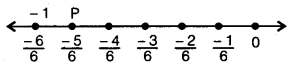Question 2.
Represent$\frac { -2 }{ 11 } ,\frac { -5 }{ 11 } ,\frac { -9 }{ 11 }$ on the number line.

Solution:
To represent$\frac { -2 }{ 11 } ,\frac { -5 }{ 11 } ,\frac { -9 }{ 11 }$ on a number line, draw a number line and mark a point O on it to represent zero. Now, mark a point P representing integers -1 on the left side of O on the number line.Divide the segment OP into eleven equal parts. Let A, B, C, D, E, F, G, H, I, J be the points of division so that OA=AB =BC = … = JP. By construction, OB is two-eleventh of OP so B represents$\frac { -2 }{ 11 }$ OE is five-eleventh of OP so E represents$\frac { -5 }{ 11 }$ and OI is nine-eleventh of OP so I represents$\frac { -9 }{ 11 }$.

Question 3.
Write five rational numbers, which are smaller than 2.

Solution:
Five rational numbers less than 2 may be taken$1,\frac { 1 }{ 2 } ,0,-1,\frac { -1 }{ 2 }$
(There can be many more such rational numbers.)

Question 4.
Find ten rational numbers between$\frac { -2 }{ 5 }$ and$\frac { 1 }{ 2 }$.

Solution:
Converting the given rational numbers with the same denominators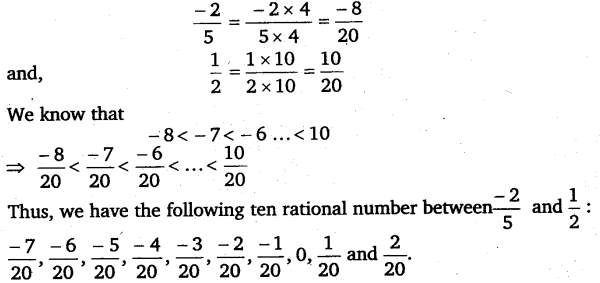Question 5.
Find five rational numbers between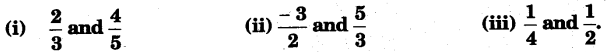Solution:
(i) Converting the given rational numbers with the same denominators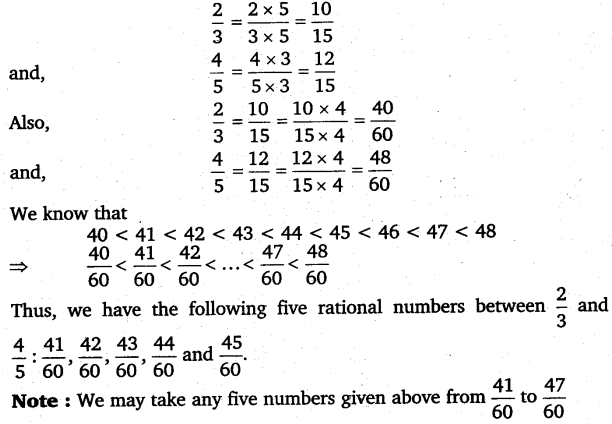(ii) Converting the given rational numbers with the same denominators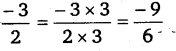(iii) Converting the given rational numbers with the same denominatorsQuestion 6.
Write five rational numbers greater than – 2.

Solution:
Five rational numbers greater than -2 may be taken as$-\frac { 3 }{ 2 } ,-1,\frac { -1 }{ 2 } ,0,\frac { 1 }{ 2 }$
There can be many more such rational numbers.

Question 7.
Find ten rational numbers between$\frac { 3 }{ 5 }$ and$\frac { 3 }{ 4 }$.

Solution:
Converting the given rational numbers with the same denominators#### Class 8 Maths NCERT Solutions for Chapter – 2 Linear Equations In One Variable Ex – 2.1

Lorem ipsum dolor sit amet, consectetur adipiscing elit. Phasellus cursus rutrum est nec suscipit. Ut et ultrices nisi. Vivamus id nisl ligula. Nulla sed iaculis ipsum.

Company Name